Examples

Chapter 10 Class 11 Straight Lines
Serial order wise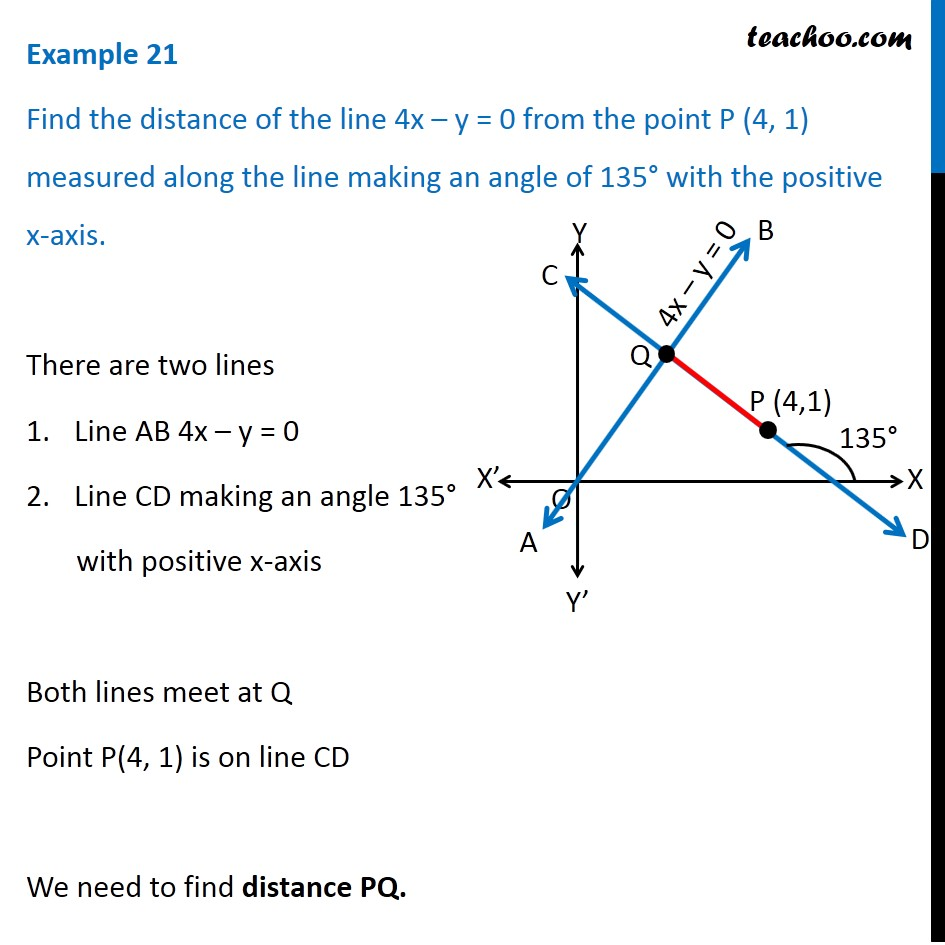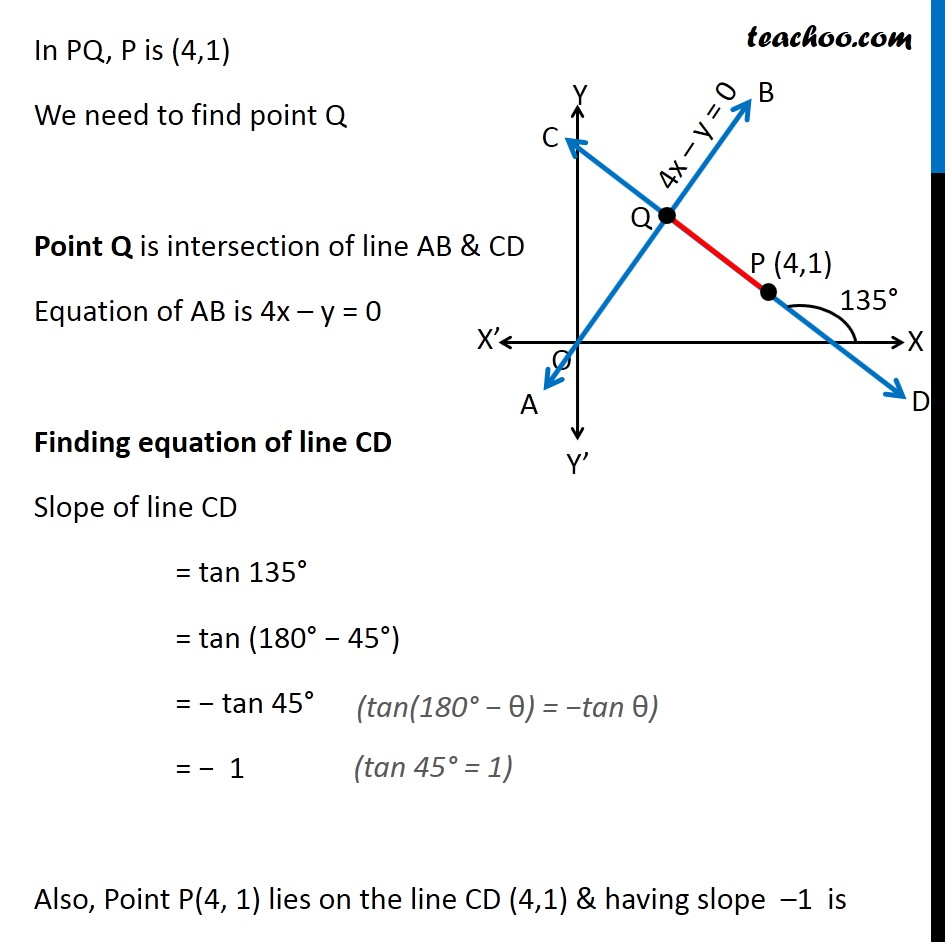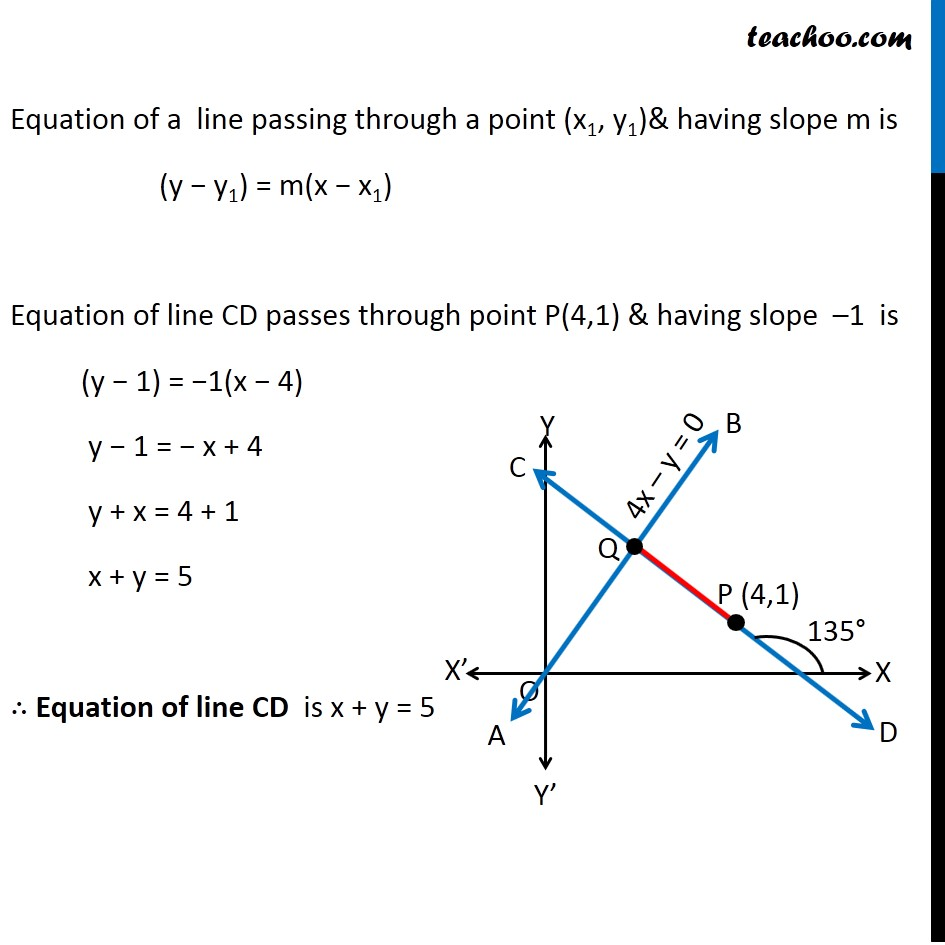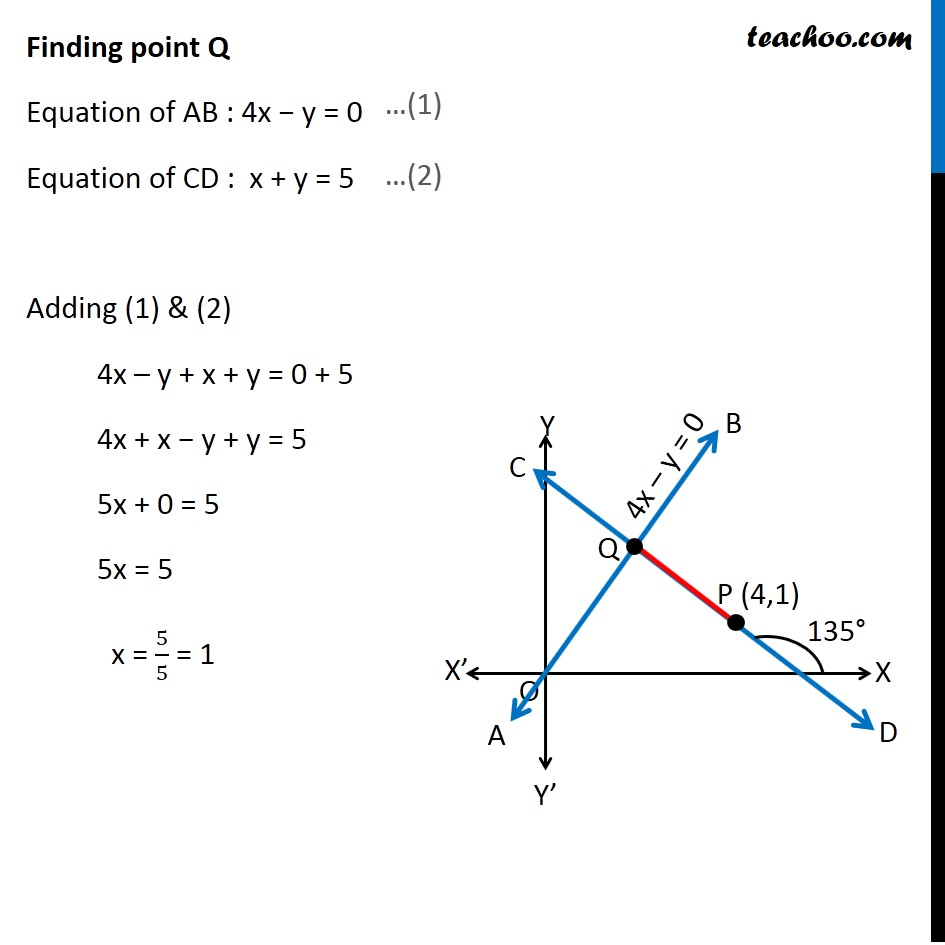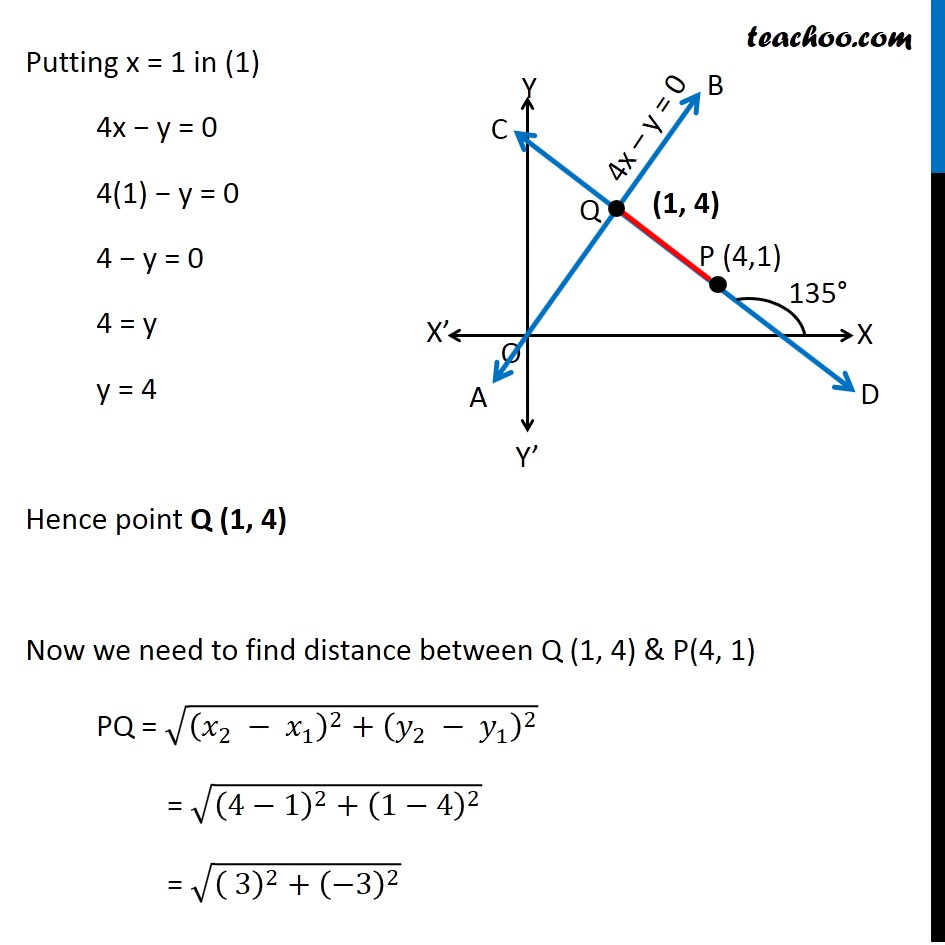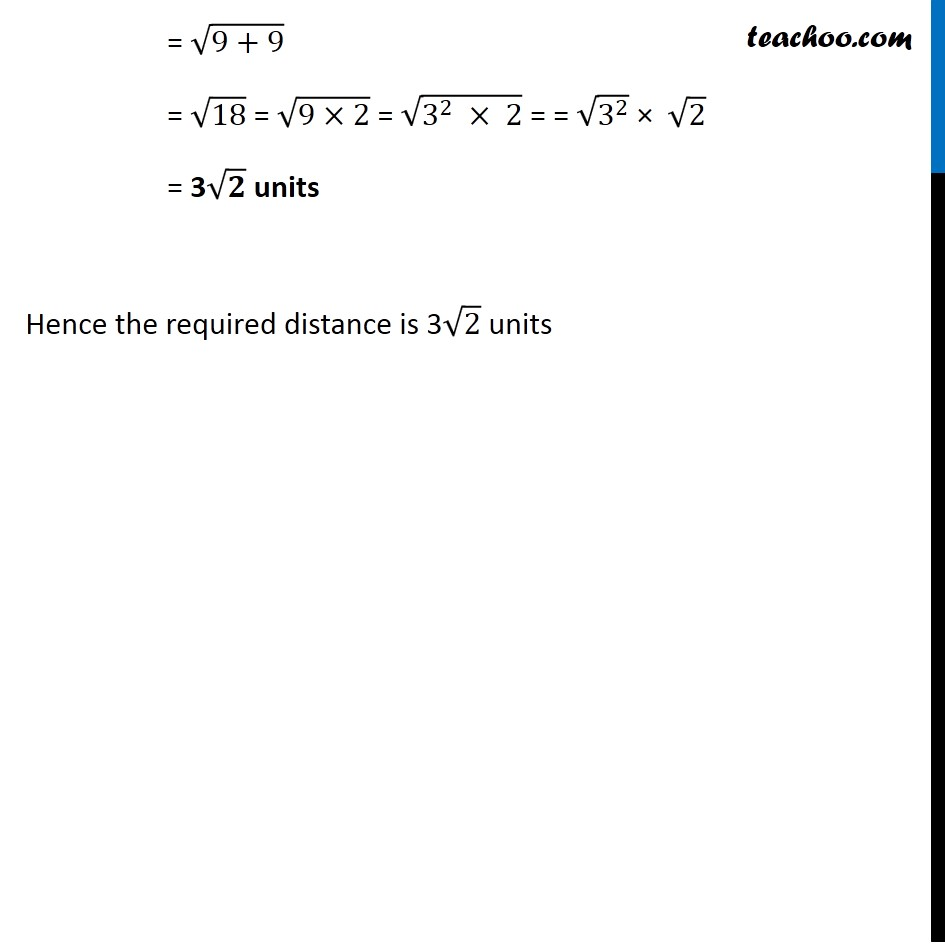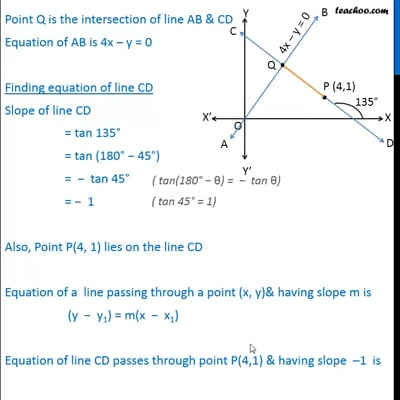This video is only available for Teachoo black users

Solve all your doubts with Teachoo Black (new monthly pack available now!)

### Transcript

Example 21 Find the distance of the line 4x – y = 0 from the point P (4, 1) measured along the line making an angle of 135° with the positive x-axis. There are two lines Line AB 4x – y = 0 Line CD making an angle 135° with positive x-axis Both lines meet at Q Point P(4, 1) is on line CD We need to find distance PQ. In PQ, P is (4,1) We need to find point Q Point Q is intersection of line AB & CD Equation of AB is 4x – y = 0 Finding equation of line CD Slope of line CD = tan 135° = tan (180° − 45°) = − tan 45° = − 1 Also, Point P(4, 1) lies on the line CD (4,1) & having slope –1 is In PQ, P is (4,1) We need to find point Q Point Q is intersection of line AB & CD Equation of AB is 4x – y = 0 Finding equation of line CD Slope of line CD = tan 135° = tan (180° − 45°) = − tan 45° = − 1 Also, Point P(4, 1) lies on the line CD (4,1) & having slope –1 is Equation of a line passing through a point (x1, y1)& having slope m is (y − y1) = m(x − x1) Equation of line CD passes through point P(4,1) & having slope –1 is (y − 1) = −1(x − 4) y − 1 = − x + 4 y + x = 4 + 1 x + y = 5 ∴ Equation of line CD is x + y = 5 Finding point Q Equation of AB : 4x − y = 0 Equation of CD : x + y = 5 Adding (1) & (2) 4x – y + x + y = 0 + 5 4x + x − y + y = 5 5x + 0 = 5 5x = 5 x = 5/5 = 1 Putting x = 1 in (1) 4x − y = 0 4(1) − y = 0 4 − y = 0 4 = y y = 4 Hence point Q (1, 4) Now we need to find distance between Q (1, 4) & P(4, 1) PQ = √((𝑥_2 − 𝑥_1 )^2+(𝑦_2 − 𝑦_1 )^2 ) = √((4−1)^2+(1−4)^2 ) = √(( 3)^2+(−3)^2 ) = √(9+9) = √18 = √(9×2) = √(3^2 × 2) = = √(3^2 ) × √2 = 3√𝟐 units Hence the required distance is 3√2 units